# NCERT Solutions Class 12 Physics Chapter-8 (Electromagnetic Waves)

NCERT Solutions Class 12 Physics from class 12th Students will get the answers of Chapter-8 (Electromagnetic Waves) This chapter will help you to learn the basics and you should expect at least one question in your exam from this chapter.
We have given the answers of all the questions of NCERT Board Physics Textbook in very easy language, which will be very easy for the students to understand and remember so that you can pass with good marks in your examination.

Class 12 Physics

Chapter-8 (Electromagnetic Waves)

Questions and answers given in practice

Chapter-8 (Electromagnetic Waves)

Question 1.

Figure shows a capacitor made of two circular plates each of radius 12 cm and separated by 5.0 cm. The capacitor is being charged by an external source (not shown in figure). The charging current is constant and equal to 0.15 A.
(a) Calculate the capacitance and the rate of change of potential difference between the plates.
(b) Obtain the displacement current across the plates.
(c) Is Kirchhoffs first rule valid at each plate of the capacitor ? Explain.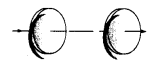Yes. Because conduction current entering one plate is equal to the displacement current leaving that plate.

Question 2.
A parallel plate capacitor (shown in the figure) made of circular plates each of radius R = 6.0 cm has a capacitance C = 100 pF. The capacitor is connected to a 230 V a.c. supply with an (angular) frequency of 300 rad s_1.
(a) What is the r .m.s. value of the conduction current?
(b) Is the conduction current equal to the displacement current?
(c) Determine the amplitude of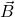at a point 3.0 cm from the axis between the plates.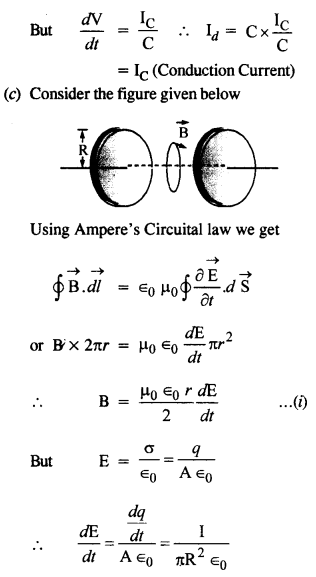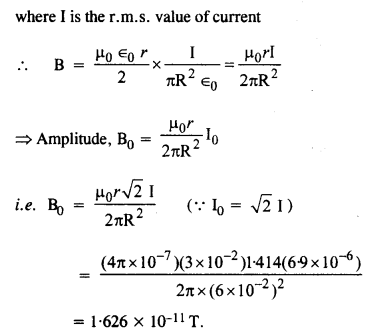Question 3.
What physical quantity is the same for X-rays of g g wavelength 10-10 m, the red light of wavelength 6800 A, and radiowaves of wavelength 500 m?

The speed in a vacuum is the same for all are c = 3 x 108 ms-1. (Electromagnetic waves)

Question 4.
A plane electromagnetic wave travels in a vacuum along Z-direction. What can you say about the directions of its electric and magnetic field vectors? If the frequency of the wave is 30 MHz, what is its wavelength?

The electric field vecto
r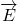and magnetic field vectorare in xy plane. They are normal to each other.

=> The corresponding wavelength band is 40 m to 25 m.

Question 6.
A charged particle oscillates about its mean equilibrium position with a frequency of 109 Hz. What is the frequency of the electromagnetic waves produced by the oscillator?
The frequency of the electromagnetic waves produced is the same as that of the oscillating charged particle. Hence the frequency of the electro­magnetic waves produced is, υ= 109 Hz.

Question 7.
The amplitude of the magnetic field part of a harmonic electromagnetic wave in a vacuum is B0 =510 nT. What is the electromagnetic waves produced by the oscillator?

Question 8.
Suppose that the electric field amplitude of an electromagnetic wave is E0 = 120 N/C and that its frequency is v = 50.0 MHz.
(a) Determine, B0, ω, k and λ.
(b) Find expressions for E and B.

Question 9.
The terminology of different parts of the electromagnetic spectrum is given in the text. Use the formula E = hv (for the energy of a quantum of radiation: photon) and obtain the photon energy in units of eV for different parts of the electromagnetic spectrum. In what way are the different scales of photon energies that you obtain related to the sources of electromagnetic radiation?

Using the relation for photon energy,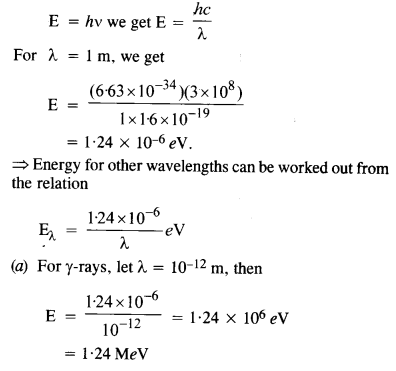The above result indicates that the different wavelengths in the electromagnetic spectrum can be obtained by multiplying roughly the powers often.
The visible wavelengths are spaced by a few eV.
The nuclear energy levels (from y rays) are spaced about 1 MeV.

Question 10.
In-plane electromagnetic wave, the electric field oscillates sinusoidally at a frequency of 2.0 x 1010 Hz and amplitude 48 Vm_1.
(a) What is the wavelength of the wave?
(b) What is the amplitude of the oscillating magnetic field?
(c) Show that the average energy density of the E field equals the average energy density of the B field. [c= 3 x 108 ms-1].

Question 11.
Suppose that the electric field part of an electromagnetic wave in a vacuum is
}.

(a) What is the direction of propagation?
(b) What is the wavelength X?
(c) What is the frequency v?
(d) What is the amplitude of the magnetic field part of the wave?
(e) Write an expression for the magnetic field part of the wave.

Question 12.
About 5% of the power of a 100 W light bulb is converted to visible radiation. What is the average intensity of visible radiation?
(a) at a distance of 1 m from the bulb?
(b) at a distance of 10 m?
Assume that the radiation is emitted isotropically and neglect reflection.

Question 13.
Use the formula λmT =
029 cm K to obtain the characteristic temperature ranges for different parts of the electromagnetic spectrum. What do the numbers that you obtain tell you?
Answer:These numbers tell us the range of temperature required to obtain the different parts of the spectrum. For example, to obtain a wavelength of 1 μm, a temperature of 2900 K is required.

Question 14.
Given below are some famous numbers associated with electromagnetic radiations in different contexts in physics. State the part of the electromagnetic spectrum to which each belongs.
(a) 21 cm (wavelength emitted by atomic hydrogen in interstellar space).
(b) 1057 MHz (frequency of radiation arising from two close energy levels in hydrogen; known as Lamb shift.)
(c) 2.7 K (temperature associated with the isotropic radiation filling all space-thought to be a relic of the ‘big-bang’ origin of the universe.)
(d) 5890 Å-5896 Å (double lines of sodium)
(e) 14.4 keV [energy of a particular transition in 57Fe nucleus associated with a famous high-resolution spectroscopic method (Mossbauer spectroscopy).]

(d) Given wavelength is of the order of 10-7 m i.e visible radiations(yellow light)

Question 15.

2. It is necessary to use satellites for long-distance TV transmission. Why?
3. Optical and radio telescopes are built on the ground but X-ray astronomy is possible only from satellites orbiting the earth. Why?
4. The small ozone layer on top of the stratosphere is crucial for human survival. Why ?
5. If the earth did not have an atmosphere, would its average surface temperature be higher or lower than what it is now?
6. Some scientists have predicted that a global nuclear war on the earth would be followed by a severe nuclear winter’ with a devastating effect on life on earth. What might be the basis of this prediction?